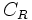# Vertical motion of a bouncing ball

This article discusses the vertical motion of a bouncing ball. The ball is either left from rest or given an initial vertical speed$u$ (upward or downward) at some initial vertical height$h$ and then left to itself. While the ball is in the air, the only force on the ball is the gravitational force, so it experiences a downward acceleration due to gravity. Each time the ball hits the floor, it bounces back with a coefficient of restitution$C_R$.